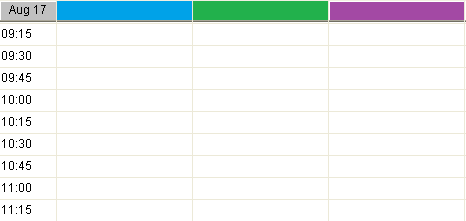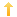## PowerBuilder – Grid datawindow object with variable number of columns

Posted on Wednesday, August 17th, 2011 at 8:20 pm in

Here is a way to build a grid datawindow which contains columns corresponding to an unknown number of data elements. You could use this approach in creating a project schedule, inventory location system, baseball box score, or any number of other examples. My example assumes the minimum number of columns to be four.

This create grid event returns a string.

```long	ll_columns, ll_count, ll_pos
string ls_errors, ls_sql, ls_dw_presentation, ls_dw_syntax, ls_find, ls_name, ls_mod_string
// base presentation information
ls_dw_presentation =    "style( type=Grid                          &
datawindow( units=1                       &
Color= 16777215)              &
column( Font.Face='Arial'         &
Font.Height=-9                    &
Font.Weight=400)                  &
text(   Font.Face='Arial' &
Font.Height=-9                    &
Font.Weight=400                   &
Border=6)"

// set initial grid to 4 columns (plus time_of_day and last_col columns)
ls_sql = "SELECT space(10) as time_of_day, space(40) as col1, space(40) as col2, space(40) as col3, space(40) as col4,"

ll_count = 5 // next column to add would be 5

// this is the variable number of data elements
ll_columns = ids_trains.rowcount()

DO WHILE ll_count <= ll_columns
ls_sql += " space(40) as col" + string(ll_count) + ","
ll_count++
LOOP

ls_sql += " '' as last_col"
ls_sql += " FROM dbo.TRAINS WHERE 1 = 2"
// build the datawindow syntax from the presentation and sql statement
ls_dw_syntax = SQLCA.Syntaxfromsql( ls_sql, ls_dw_presentation, ls_errors)
IF Len(ls_errors) = 0 THEN
// widen the header band a bit
// create dwo and assign to grid
dw_grid.create(ls_dw_syntax,ls_errors)
END IF
// appearance modifies
dw_grid.modify("last_col.visible=FALSE")

FOR ll_count = 1 TO Long(dw_grid.Object.DataWindow.Column.Count)
IF dw_grid.Describe("#" + String(ll_count) + ".Visible") = "1" Then
ls_name = dw_grid.Describe("#" + String(ll_count) + ".Name")
ls_mod_string = ls_name + "_t.background.mode=0" // must set to opaque or color does not show
ls_errors = dw_grid.modify(ls_mod_string)
// set the column heading color
ls_mod_string = ls_name + "_t.background.color=12632256"
ls_errors = dw_grid.modify(ls_mod_string)
IF POS(ls_name ,'time_of_day') = 0 THEN
ls_mod_string = ls_name + "_t.Alignment=0" //left
ls_errors = dw_grid.modify(ls_mod_string)
END IF
ls_mod_string = ls_name + "_t.Height=18"
ls_errors = dw_grid.modify(ls_mod_string)
ls_mod_string = ls_name + "_t.tooltip.enabled=1"
ls_errors = dw_grid.modify(ls_mod_string)
END IF
NEXT
RETURN ls_errors```

Now I know you can do several modifies with a single statement but it is much easier to debug if you do a single attribute at a time.

Once you have the grid set up you can call subsequent methods to populate it (and make columns invisible if needed), resize the width of the columns, etc.Example grid for a time schedule with fifteen minute increments.

You might also be interested in
Tags: